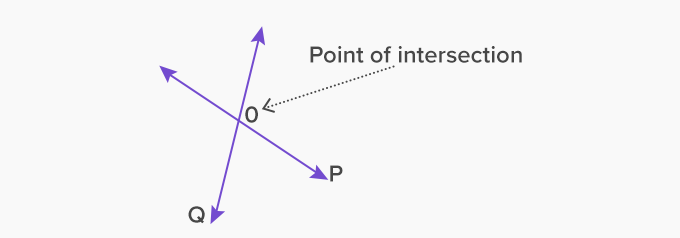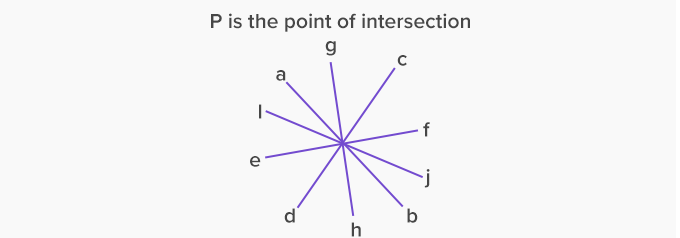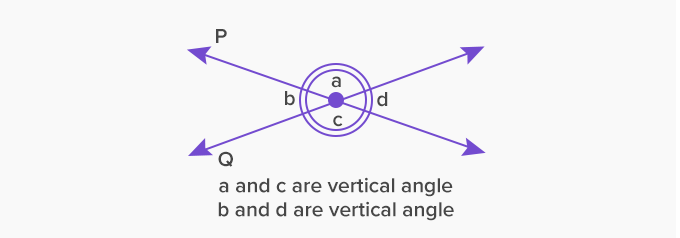# Intersecting Lines - Definition with Examples

The Complete K-5 Math Learning Program Built for Your Child

• 30 Million Kids

Loved by kids and parent worldwide

• 50,000 Schools

Trusted by teachers across schools

• Comprehensive Curriculum

Aligned to Common Core

What is Intersecting Lines?

When two or more lines cross each other in a plane, they are called intersecting lines. The intersecting lines share a common point, which exists on all the intersecting lines, and is called the point of intersection.

Here, lines P and Q intersect at point O, which is the point of intersection.In the given image below, there are many straight lines crossing each other and intersecting at the common point P.Properties of intersecting lines

• The intersecting lines (two or more) meet only at one point always.

• The intersecting lines can cross each other at any angle. This angle formed is always greater than 0o  and less than 180o .

• Two intersecting lines form a pair of vertical angles. The vertical angles are opposite angles with a common vertex (which is the point of intersection).

Here, ∠a and ∠c are vertical angles and are equal.  Also, ∠b and ∠d are vertical angles and equal to each other.

∠a + ∠d = straight angle = 180°Intersecting lines in real life